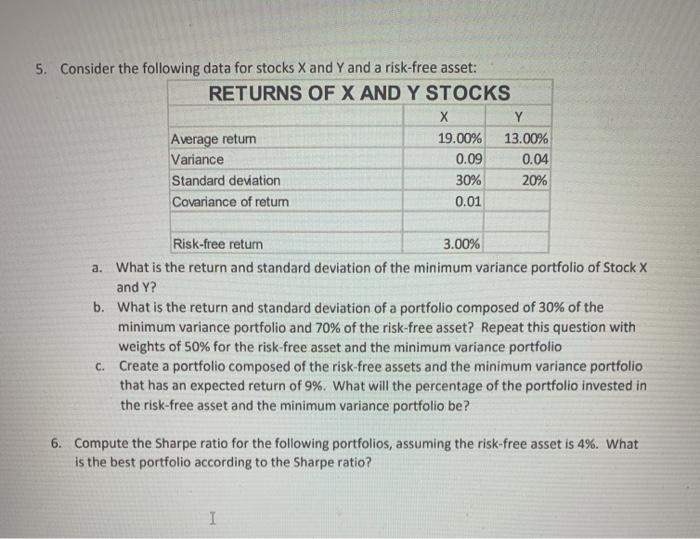# Question 5. Consider the following data for stocks X and Y and a risk-free asset: RETURNS OF X AND Y STOCKS х Y Average return 19.00% 13.00% Variance 0.09 0.04 Standard deviation 30% 20% Covariance of retum 0.01 Risk-free return 3.00% a. What is the return and standard deviation of the minimum variance portfolio of Stock X and Y? b. What is the return and standard deviation of a portfolio composed of 30% of the minimum variance portfolio and 70% of the risk-free asset? Repeat this question with weights of 50% for the risk-free asset and the minimum variance portfolio c. Create a portfolio composed of the risk-free assets and the minimum variance portfolio that has an expected return of 9%. What will the percentage of the portfolio invested in the risk-free asset and the minimum variance portfolio be? 6. Compute the Sharpe ratio for the following portfolios, assuming the risk-free asset is 4%. What is the best portfolio according to the Sharpe ratio? I